# Use Case 3: Hydrographic Statistics

In this example, we compute some useful hydrographic statistics. We show how to properly account for different grid cell volumes using weighted statistics. The computation is accelerated using dask.

:

# Setup environment:
import matplotlib.pyplot as plt

import numpy as np
import xarray as xr

import oceanspy as ospy


The following cell starts a dask client (see the Dask Client section in the tutorial).

:

import warnings

# Start client for multiprocessing

client = Client()
client

:


### Client

Client-26ae3825-d8e2-11ed-8cff-0242ac110006

 Connection method: Cluster object Cluster type: distributed.LocalCluster Dashboard: http://127.0.0.1:8787/status

### Cluster Info

Open the oceandataset and compute the potential density anomaly:

:

SciServer = True  # True: SciServer - False: any computer
if SciServer:
od_snapshot = ospy.open_oceandataset.from_catalog("get_started")
else:
import os

if not os.path.isdir("oceanspy_get_started"):
import subprocess

print("...it might take a couple of minutes.")
commands = [
"wget -v -O oceanspy_get_started.tar.gz -L "
"https://livejohnshopkins-my.sharepoint.com/"
":u:/g/personal/malmans2_jh_edu/"
"EXjiMbANEHBZhy62oUDjzT4BtoJSW2W0tYtS2qO8_SM5mQ?"
"tar xvzf oceanspy_get_started.tar.gz",
"rm -f oceanspy_get_started.tar.gz",
]
subprocess.call("&&".join(commands), shell=True)
od_snapshot = ospy.open_oceandataset.from_zarr("oceanspy_get_started")

od_snapshot = od_snapshot.compute.potential_density_anomaly()

Opening get_started.
Small cutout from EGshelfIIseas2km_ASR_crop.
Citation:
* Almansi et al., 2020 - GRL.
* EGshelfIIseas2km_ASR_full: Full domain without variables to close budgets.
* EGshelfIIseas2km_ASR_crop: Cropped domain with variables to close budgets.
Computing potential density anomaly using the following parameters: {'eq_state': 'jmd95'}.


Make a temperature/salinity diagram.

:

# The statistics are computed using these data.
fig = plt.figure(figsize=(10, 10))
od_snapshot.plot.TS_diagram(markersize=1, alpha=0.5)

# Print number of samples
n_samples = od_snapshot.dataset["Temp"].count().values
print("\nNumber of samples: {:,}\n".format(n_samples))

Isopycnals: Computing potential density anomaly using the following parameters: {'eq_state': 'jmd95'}.

Number of samples: 5,927,388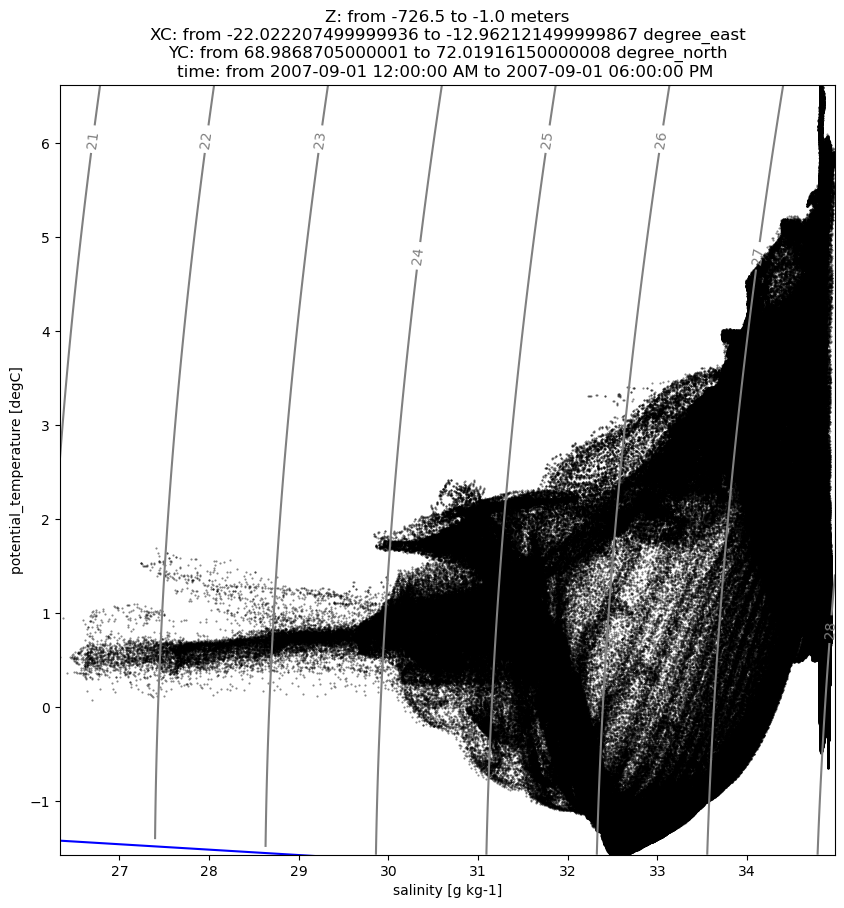The temperature/salinity diagram shows the distribution of watermass types in terms of their hydrographic properties. Each dot represents a single grid cell in the dataset. We will compute statistics using this data to interpret the plot above.

## Binning and Parallelization

We define a function bin_snapshot to perform the binning we need to compute the volume-weighted statistics, or the mode of a variable. The use of the xarray stack method accelerates the groupby_bins task.

:

# Function for binning a single snapshot in time
# dat       = data array to bin (e.g., temperature distributed over 3D space)
# weights   = data array of the relative volumes of the cells in 3D space
# bin_edges = array of the edges of the bins (e.g. temperature bins)
# bin_mid   = array of the mid-points of the bins

def bin_snapshot(dat, weight, bin_edges, bin_mid):
# Speed up by stacking over space
dat = dat.stack(alldims=["X", "Y", "Z"])
weight = weight.stack(alldims=["X", "Y", "Z"])

# Bin the weight data into these variable bins
# using the xarray groupby_bins method
return weight.groupby_bins(
dat.rename("groups"),
bins=bin_edges,
labels=bin_mid,
include_lowest=True,
).sum()


Pick True or False for the parallel variable below to run bin_snapshot with/without dask.

:

# Parallel switch
parallel = True


## Raw statistics

First compute and report some raw statistics using xarray, for comparison below. Because we ignore the different volumes of model grid cells, these statistics are not representative of the underlying fields.

:

# Compute and print raw statistics

# Define the percentiles required:
qiles = [0.01, 0.50, 0.99]

# Define the variables required:
var_list = ["Temp", "S", "Sigma0"]

# Pretty print the results:
print("Raw statistics (not volume-weighted):")
print(
"{:>25s}: {:>10s} {:>10s} {:>10s} {:>10s} {:>10s} {:>10s} {:s}".format(
"Variable", "mean", "std", "mode", "1", "50", "99", "percentiles"
)
)

# Loop over variables computing statistics using xarray methods.
# Variables need to be load because dask chunks are not compatible
# with xarray quantiles function.
for var in var_list:
print("{:25s}:".format(od_snapshot.dataset[var].long_name), end=" ")

# Data

# Mean
var_mean = dats.mean(keep_attrs=True)
print("{:10.4g}".format(var_mean.values), end=" ")

# Std
var_std = dats.std(keep_attrs=True)
print("{:10.4g}".format(var_std.values), end=" ")

# Mode
bin_edges = np.linspace(dats.min(), dats.max(), 512)
bin_mid = (bin_edges[:-1] + bin_edges[1:]) / 2
# Parallel switch
if parallel:

dat1 = [
bin_snapshot_(
bin_edges,
bin_mid,
)
for time in dats["time"]
]

with warnings.catch_warnings():
warnings.simplefilter("ignore")
else:
dat1 = [
bin_snapshot(
dats.sel(time=time),
xr.ones_like(dats).sel(time=time),
bin_edges,
bin_mid,
)
for time in dats["time"]
]
dat1 = xr.concat(dat1, "time").sum("time")
var_mode = dat1["groups_bins"].where(dat1 == dat1.max(), drop=True)
print("{:10.4g}".format(var_mode.values.squeeze()), end=" ")

# Qiles
var_qiles = dats.quantile(qiles, keep_attrs=True)
print(
"{:10.4g} {:10.4g} {:10.4g} {:s}".format(
var_qiles.sel(quantile=qiles).values,
var_qiles.sel(quantile=qiles).values,
var_qiles.sel(quantile=qiles).values,
od_snapshot.dataset[var].units,
)
)

del dats

Raw statistics (not volume-weighted):
Variable:       mean        std       mode          1         50         99 percentiles
potential_temperature    :     0.8009      1.366     0.1019     -1.421     0.4803      5.716 degC
salinity                 :      34.44     0.9865       34.9      30.68      34.89      34.93 g kg-1
potential density anomaly:      27.59     0.7954      28.01      24.55      27.97      28.05 kg/m^3


## Weighted statistics

Compute the weighted mean (OceanSpy provides a method to do so).

:

od_snapshot = od_snapshot.compute.weighted_mean(varNameList=var_list)

# Print computed variables
for var in od_snapshot.dataset.data_vars:
if any(
[(var == v or var == f"weight_{v}" or var == f"w_mean_{v}") for v in var_list]
):
print(
"{:>15}: {} [{}]".format(
var,
od_snapshot.dataset[var].long_name,
od_snapshot.dataset[var].units,
)
)

Computing weighted_mean.

Temp: potential_temperature [degC]
S: salinity [g kg-1]
Sigma0: potential density anomaly [kg/m^3]
w_mean_Temp: potential_temperature [degC]
weight_Temp: Weights for average [s*m^2*m]
w_mean_S: salinity [g kg-1]
weight_S: Weights for average [s*m^2*m]
w_mean_Sigma0: potential density anomaly [kg/m^3]
weight_Sigma0: Weights for average [s*m^2*m]


The weights used by OceanSpy have been stored in od_snapshot. The weights correspond to space-time volume elements $$dx \; dy\; dz\; dt$$ and hence have units of m$$^3$$s. The variations in the weights are dominated by variations in grid cell volume, which comes from $$dz$$ (see below), although $$dx$$ and $$dy$$ are also important. As the timestep $$dt$$ is constant it isn’t responsible for any variation in the weights. We can use the weights, for example, to color a TS-diagrams and better understand which temperature and salinity values are misrepresented using raw statistics.

:

# Scatter plots are not optimized for large amount of samples,
# so the following TS-diagram only shows sea surface water.
fig = plt.figure(figsize=(10, 10))
ax = od_snapshot.plot.TS_diagram(
s=1,
alpha=0.5,
colorName="weight_Temp",
cutout_kwargs={"ZRange": 0, "dropAxes": True},
)

Cutting out the oceandataset.
Isopycnals: Computing potential density anomaly using the following parameters: {'eq_state': 'jmd95'}.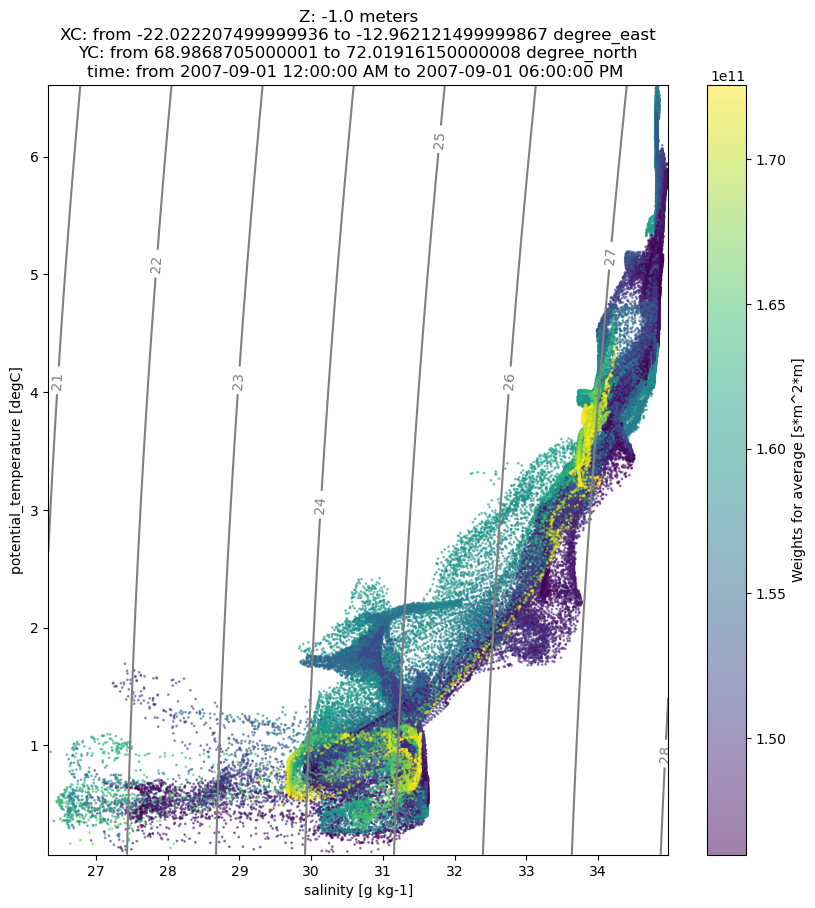The vertical resolution of the model linearly increases from 1 to 15m in the upper 120m and is 15m thereafter. The following plots show that the weights of warmer and fresher water masses are overestimated in the raw statistics.

:

fig = plt.figure(figsize=(10, 10))

for i, (var, pos) in enumerate(zip(["Temp", "S"], [221, 223])):
ax = plt.subplot(pos)
data = od_snapshot.dataset[var]
weight = od_snapshot.dataset[f"weight_{var}"]
ax.plot(
data.values.flatten(),
weight.values.flatten(),
".",
color=f"C{i}",
alpha=0.5,
markersize=0.5,
)
ax.set_xlabel(f"{data.long_name} [{data.units}]")
ax.set_ylabel(f"{weight.long_name} [{weight.units}]")
ax = plt.subplot(122)
drF = od_snapshot.dataset["drF"]
Z = od_snapshot.dataset["Z"]
line = ax.plot(drF, Z, ".-", color="k", linewidth=3, markersize=15)
xlab = ax.set_xlabel(f"{drF.long_name} [{drF.units}]")
ylab = ax.set_ylabel(f"{Z.long_name} [{Z.units}]")
tit = ax.set_title("Model vertical resolution")
ax.grid(True)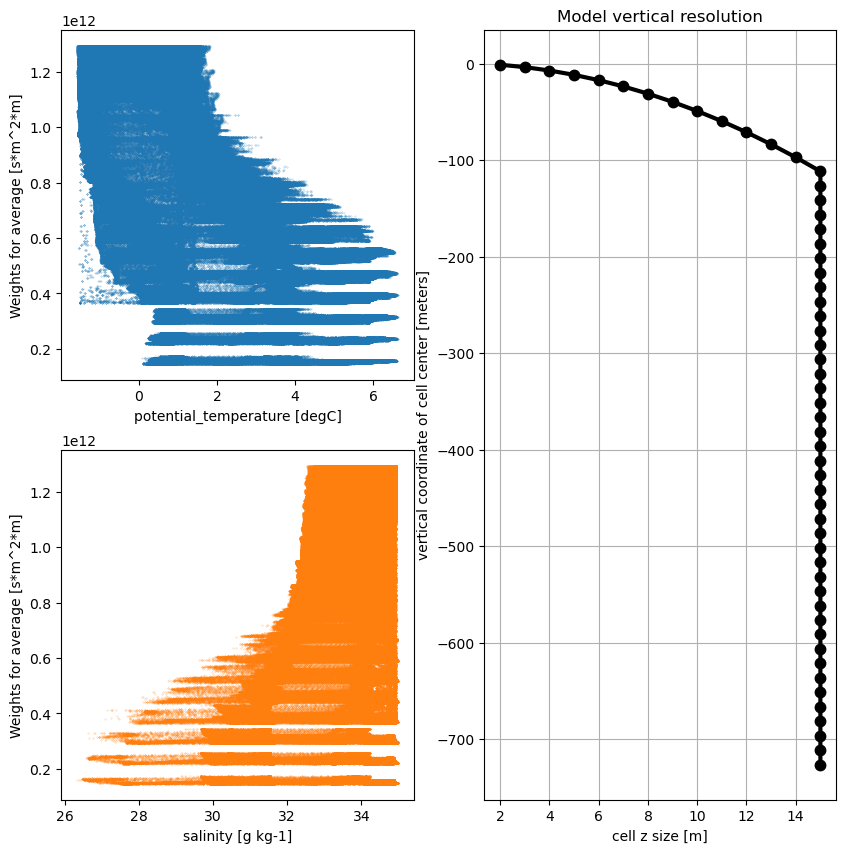Next compute and report the same statistics as before but taking into account the different volumes of different grid cells. We also plot probability distribution and cumulative distribution functions.

:

# Setup plotting
fig, axes = plt.subplots(1, len(var_list), figsize=(30, 10))
hist_color = "tab:red"
qiles_color = "tab:blue"
stat_color = "tab:green"
stat_color2 = "tab:cyan"
stat_color3 = "tab:pink"
stat_color4 = "tab:brown"
bin_edges2 = np.linspace(0, 1, 512)
qiles2 = (bin_edges2[:-1] + bin_edges2[1:]) / 2

# Pretty print the results:
print("Raw statistics (not volume-weighted):")
print(
"{:>25s}: {:>10s} {:>10s} {:>10s} {:>10s} {:>10s} {:>10s} {:s}".format(
"Variable", "mean", "std", "mode", "1", "50", "99", "percentiles"
)
)

# Loop over variables of interest to compute std and percentiles (quantiles),
# pretty print, and plot
for i, (var, ax) in enumerate(zip(var_list, axes.flat)):
# Print on the way to monitor progress
var_name = od_snapshot.dataset[var].long_name
units = od_snapshot.dataset[var].attrs["units"]
print("{:25s}:".format(var_name), end=" ")

# Extract variables
dats = getattr(od_snapshot.dataset, var)
weights = getattr(od_snapshot.dataset, "weight_" + var)

# Normalize weights
weights = weights.where(dats.notnull())
weightsum = weights.sum()
weights = weights / weightsum

# Compute the arithmetic mean
var_mean = getattr(od_snapshot.dataset, "w_mean_" + var)
print("{:10.4g}".format(var_mean.values), end=" ")

# There is no OceanSpy method to compute
# the weighted standard deviation or percentiles.

# Compute the standard deviation
dat2 = ((od_snapshot.dataset[var] - var_mean) ** 2) * weights
var_std = np.sqrt(dat2.sum().values)
print("{:10.4g}".format(var_std), end=" ")

# Define bin edges and mid points for the variable in question.
# We use 512 uniformly-spaced bins:
bin_edges = np.linspace(dats.min(), dats.max(), 512)
bin_mid = (bin_edges[:-1] + bin_edges[1:]) / 2

# Compute percentiles:
# Parallel switch
if parallel:
dat3 = [
bin_snapshot_(
bin_edges,
bin_mid,
)
for time in dats["time"]
]
with warnings.catch_warnings():
warnings.simplefilter("ignore")
else:
dat3 = [
bin_snapshot(
dats.sel(time=time), weights.sel(time=time), bin_edges, bin_mid
)
for time in dats["time"]
]

# Concatenate dat
dat3 = xr.concat(dat3, "time").sum("time")
var_hist = dat3 / weights.sum()
dat4 = dat3.cumsum()
dat5 = dat4 / weights.sum()

# Mode
var_mode = dat3["groups_bins"].where(dat3 == dat3.max(), drop=True)
print("{:10.4g}".format(var_mode.values.squeeze()), end=" ")

# Use numpy interpolation to find the percentiles
# - effectively evaluating the inverse CDF
var_qiles = np.interp(qiles, dat5, bin_mid)
var_qiles2 = np.interp(qiles2, dat5, bin_mid)
var_median = np.interp(0.50, dat5, bin_mid)
var_limits = np.interp([0.01, 0.99], dat5, bin_mid)
for var_qile in var_qiles:
print("{:10.4g}".format(var_qile), end=" ")
print("{:s}".format(units))

# Plot the PDF
p0 = ax.plot(bin_mid, var_hist, "-", color=hist_color, label="PDF", linewidth=4)
ax.set_xlabel(var_name + " [" + units + "]", fontsize=15)
ax.set_ylabel("Probability distribution function", fontsize=15, color=hist_color)
ax.tick_params(axis="y", labelcolor=hist_color)
ax.grid(True)
ax.set_xlim(var_limits)

# Compute and plot the CDF
ax2 = ax.twinx()
p1 = ax2.plot(var_qiles2, qiles2, "-", color=qiles_color, label="CDF", linewidth=4)
ax2.set_ylabel("Cumulative distribution function", fontsize=15, color=qiles_color)
ax2.tick_params(axis="y", labelcolor=qiles_color)
ax2.set_xlim(var_limits)

p2 = ax.axvspan(var_mean - var_std, var_mean + var_std, color=stat_color)
l1 = ax.axvline(var_mean, linestyle="-", color="w", linewidth=2)
l2 = ax.axvline(var_median, linestyle="--", color=stat_color3, linewidth=2)
l3 = ax.axvline(var_mode, linestyle="--", color=stat_color4, linewidth=2)
ax.legend((l2, l3, p2), ("median", "mode", "mean +/- std"), loc="upper left")

Raw statistics (not volume-weighted):
Variable:       mean        std       mode          1         50         99 percentiles
potential_temperature    :     0.5422      1.033     0.1019     -1.435     0.3659      4.811 degC
salinity                 :       34.6     0.7318       34.9       31.5      34.89      34.92 g kg-1
potential density anomaly:      27.75     0.5858      28.01      25.24      27.98      28.05 kg/m^3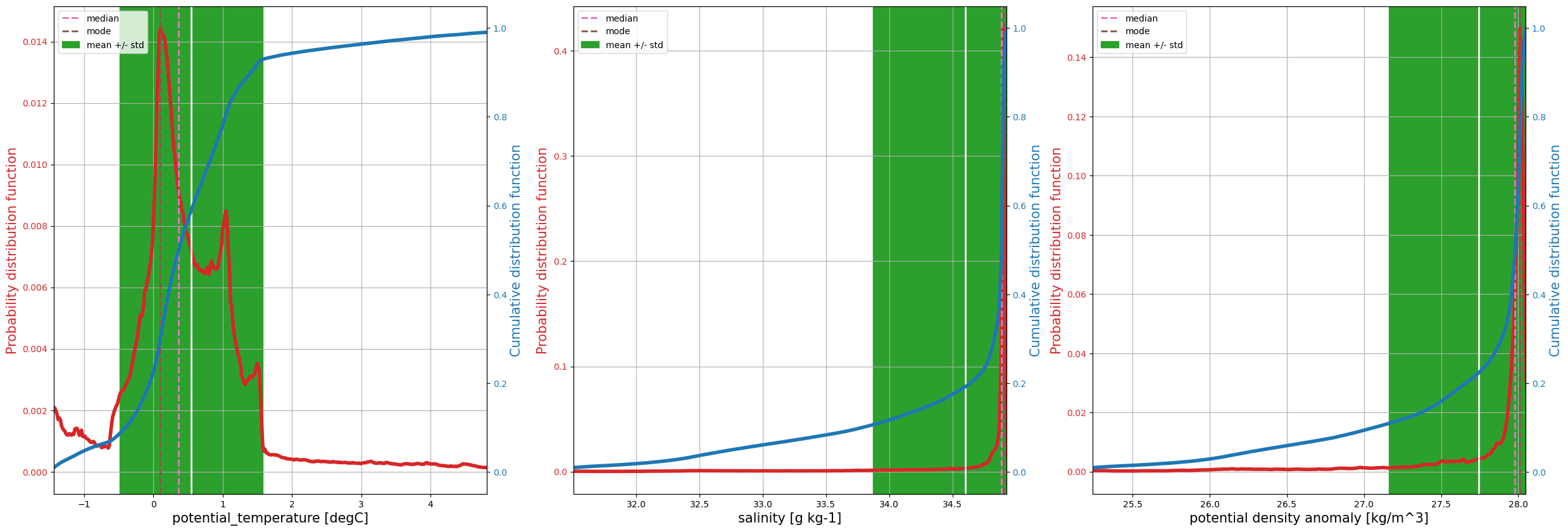Notice the difference between the weighted mean statistics (in the table of numbers immediately above) and the raw statistics (further above). For example, the weighted-mean temperatures are significantly cooler. The reason is that the grid cell volumes vary, which is ignored in the computation of the raw mean.

Notice how the salinity distribution (probability density function) resembles the potential density anomaly distribution, indicating that density is controlled by salinity, not temperature. This fact is reflected in the T/S diagrams above by the nearly vertical contours of density anomaly. This is a beta-ocean in the parlance of Carmack (2007) and Stewart & Haine (2016).

Notice how the computation is accelerated using dask (try running with/without dask by setting the parallel flag at the top of this notebook).

## Volumetric census

A natural extension of these concepts is to compute and plot the volumetric census over temperature/salinity classes. The plots above of probability distribution and cumulative distribution functions focus only on the variable of interest. In other words, the temperature binning to construct the histogram (var_hist in the code) ignores the salinity. But we’re also interested in binning simultaneously into temperature and salinity classes. This quantity is (essentially) the joint distribution function of seawater volume over temperature and salinity.

To compute the joint distribution of volume over temperature and salinity requires two-dimensional binning. This functionality is achieved using the xhistogram package.

:

from xhistogram.xarray import histogram


First we construct a histogram of the number of data points in each temperature and salinity class. The temperature and salinity classes are defined using tbins and sbins. All timesteps are considered in the histogram. The colorscale is logarithmic.

:

# Histogram of number of data points. From xhistogram tutorial:
sbins = np.arange(25.5, 35.5, 0.0125)
tbins = np.arange(-2, 10, 0.05)
fig = plt.figure(figsize=(10, 6))
hTS = histogram(
od_snapshot.dataset["S"].mean("time"),
od_snapshot.dataset["Temp"].mean("time"),
bins=[sbins, tbins],
)
np.log10(hTS.T).plot(levels=31)

/home/idies/mambaforge/envs/Oceanography/lib/python3.9/site-packages/dask/core.py:119: RuntimeWarning: divide by zero encountered in log10
return func(*(_execute_task(a, cache) for a in args))

:

<matplotlib.collections.QuadMesh at 0x7f82773b34f0>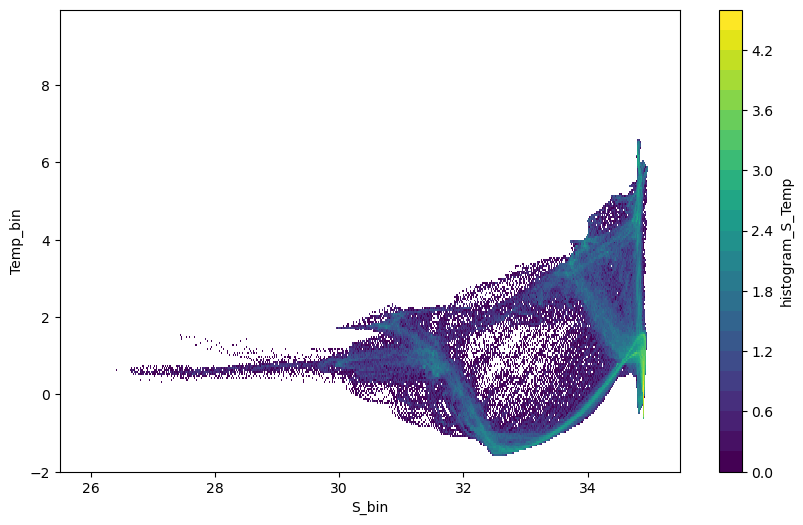Next we construct the histogram taking into account the different cell volumes. The weight_S variable from compute.weighted_mean supplies these cell volumes (we could also use the weight_T variable). This histogram shows the volume ($$m^3$$) in each temperature and salinity class.

:

# Histogram using volume weights.
dVol = ospy.compute.weighted_mean(
od_snapshot, varNameList="S", axesList=["X", "Y", "Z"]
)["weight_S"]
fig = plt.figure(figsize=(10, 6))
hTSw = histogram(
od_snapshot.dataset["S"],
od_snapshot.dataset["Temp"],
bins=[sbins, tbins],
weights=dVol,
)
np.log10(hTSw.T).plot(levels=31)

Computing weighted_mean.

/home/idies/mambaforge/envs/Oceanography/lib/python3.9/site-packages/dask/core.py:119: RuntimeWarning: divide by zero encountered in log10
return func(*(_execute_task(a, cache) for a in args))

:

<matplotlib.collections.QuadMesh at 0x7f819f416d30>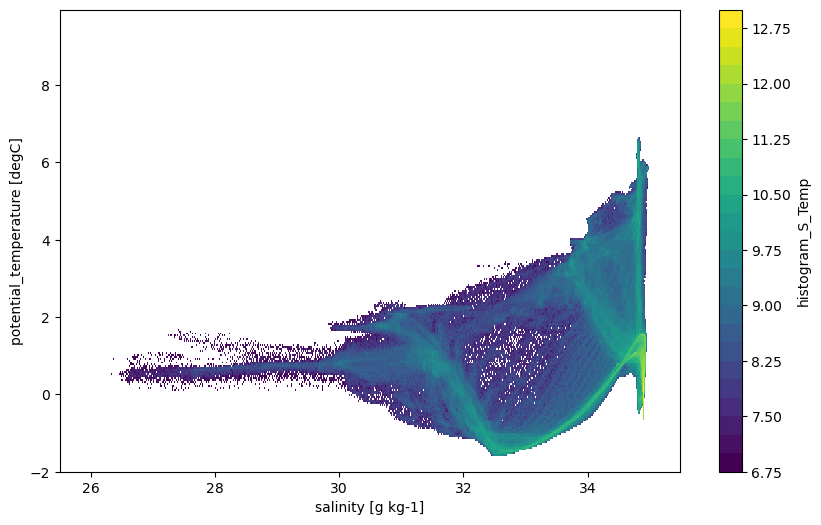This is the volumetric census over water temperature and salinity classes.